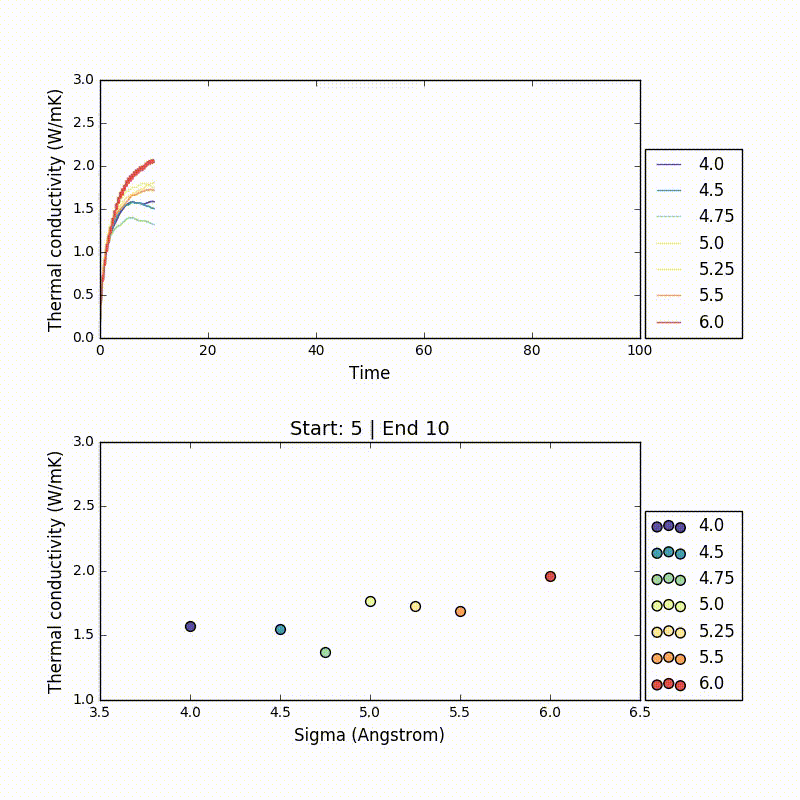# TherMOF

Investigating thermal transport in Metal-Organic Frameworks

KBS - Home
TherMOF
Methods
Results

## Estimating thermal conductivity

Estimating a thermal conductivity (k) value from NVE simulations can be tricky. After the autocorrelation function is calculated, k is estimated as the converging value. However, if sampling is not enough the autocorrelation function might not converge in the given time frame. As a result, it is not easy to estimate the k automatically without visual inspection. Here is an animation showing the variability of k according to how it’s estimated.In the plot above the thermal conductivity autocorellation functions are showed and in the bottom plot a k is estimated by taking the average between start and end timesteps. According to where the average is taken the trends in the plot change dramatically.